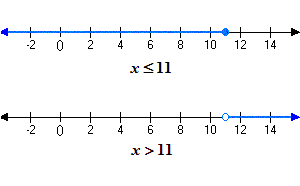# Graphing Inequalities in One Variable

The solutions of inequalities can be graphed on the number line as rays. If the inequality is "strict" ( $<$ or $>$ ), we use an open dot to indicate that the endpoint of the ray is not part of the solution. For the other types of inequalities ( $\le$ and $\ge$ ), we use a closed dot .

Example: